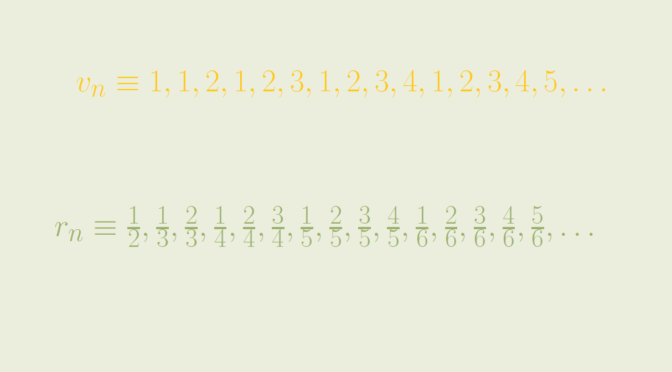Limit points of real sequences

Let’s start by recalling an important theorem of real analysis:

THEOREM. A necessary and sufficient condition for the convergence of a real sequence is that it is bounded and has a unique limit point.

As a consequence of the theorem, a sequence having a unique limit point is divergent if it is unbounded. An example of such a sequence is the sequence $u_n = \frac{n}{2}(1+(-1)^n),$ whose initial values are $0, 1, 0, 2, 0, 3, 0, 4, 0, 5, 6, \dots$ $$(u_n)$$ is an unbounded sequence whose unique limit point is $$0$$.

Let’s now look at sequences having more complicated limit points sets.

A sequence whose set of limit points is the set of natural numbers

Consider the sequence $$(v_n)$$ whose initial terms are $1, 1, 2, 1, 2, 3, 1, 2, 3, 4, 1, 2, 3, 4, 5, \dots$ $$(v_n)$$ is defined as follows $v_n=\begin{cases} 1 &\text{ for } n= 1\\ n – \frac{k(k+1)}{2} &\text{ for } \frac{k(k+1)}{2} \lt n \le \frac{(k+1)(k+2)}{2} \end{cases}$ $$(v_n)$$ is well defined as the sequence $$(\frac{k(k+1)}{2})_{k \in \mathbb N}$$ is strictly increasing with first term equal to $$1$$. $$(v_n)$$ is a sequence of natural numbers. As $$\mathbb N$$ is a set of isolated points of $$\mathbb R$$, we have $$V \subseteq \mathbb N$$, where $$V$$ is the set of limit points of $$(v_n)$$. Conversely, let’s take $$m \in \mathbb N$$. For $$k + 1 \ge m$$, we have $$\frac{k(k+1)}{2} + m \le \frac{(k+1)(k+2)}{2}$$, hence $u_{\frac{k(k+1)}{2} + m} = m$ which proves that $$m$$ is a limit point of $$(v_n)$$. Finally the set of limit points of $$(v_n)$$ is the set of natural numbers.

A sequence whose set of limit points is the segment $$[0,1]$$

Taking advantage of the sequence $$(v_n)$$, let’s now consider $$(r_n)$$ whose initial terms are $\frac{1}{2}, \frac{1}{3}, \frac{2}{3}, \frac{1}{4}, \frac{2}{4}, \frac{3}{4}, \frac{1}{5}, \frac{2}{5}, \frac{3}{5}, \frac{4}{5}, \frac{1}{6}, \frac{2}{6}, \frac{3}{6}, \frac{4}{6}, \frac{5}{6}, \dots$ Formal definition of $$(r_n)$$ is $r_n=\begin{cases} \frac{1}{2} &\text{ for } n= 1\\ \frac{n}{k+2} – \frac{k(k+1)}{2(k+2)} &\text{ for } \frac{k(k+1)}{2} \lt n \le \frac{(k+1)(k+2)}{2} \end{cases}$ The values of the sequence $$(r_n)$$ are in $$(0,1) \cap \mathbb Q$$. Moreover, one can notice that $$(r_n)$$ takes each rational number of $$(0,1)$$ as value an infinite number of times. Indeed for $$\frac{p}{q} \in (0,1)$$ with $$1 \le p \lt q$$ and $$m \ge 1$$ we have $\frac{(mq-2)(mq-1)}{2} \lt \frac{(mq-2)(mq-1)}{2} + mp$ and \begin{aligned} \frac{(mq-2)(mq-1)}{2} + mp &\le \frac{(mq-2)(mq-1)}{2} + m(q-1)\\ &\le \frac{(mq-2)(mq-1)}{2} + mq-1\\ &= \frac{(mq-1)mq}{2} \end{aligned} Hence \begin{aligned} r_{\frac{(mq-2)(mq-1)}{2} + mp} &= \frac{(mq-2)(mq-1)}{2mq} + \frac{mp}{mq} – \frac{(mq-2)(mq-1)}{2mq}\\ &= \frac{mp}{mq}\\ &= \frac{p}{q} \end{aligned} proving the desired result. As the rational numbers of the segment $$(0,1)$$ are dense in $$[0,1]$$, we can conclude that the set of limit points of $$(r_n)$$ is exactly the interval $$[0,1]$$.

A sequence whose set of limit points is $$\mathbb R$$

Consider the real function $\begin{array}{l|rcll} f : & (0,1) & \longrightarrow & \mathbb R\\ & x & \longmapsto & \frac{2x-1}{x(1-x)} \end{array}$ One can verify that $$f$$ is continuous, strictly increasing and $\lim\limits_{x \to 0^+} f(x) = -\infty, \ \lim\limits_{x \to 1^-} f(x) = +\infty.$ Therefore $$f$$ is a bijection from $$(0,1)$$ onto $$\mathbb R$$. We claim that the set of limit points of the rational sequence $$(f(r_n))$$ is $$\mathbb R$$.

For $$y_0 \in \mathbb R$$, let’s take the unique $$x_0 \in (0,1)$$ such that $$f(x_0)=y_0$$. As the set of limit points of $$(r_n)$$ is $$[0,1]$$, one can find a subsequence $$(r_{j(n)})$$ converging to $$x_0$$. $$f$$ being continuous, the sequence $$(f(r_{j(n)}))$$ converges to $$f(x_0)=y_0$$, concluding our proof.